Courses

# Test: Stresses in Beams Level - 1

## 20 Questions MCQ Test Strength of Materials (SOM) | Test: Stresses in Beams Level - 1

Description
This mock test of Test: Stresses in Beams Level - 1 for Mechanical Engineering helps you for every Mechanical Engineering entrance exam. This contains 20 Multiple Choice Questions for Mechanical Engineering Test: Stresses in Beams Level - 1 (mcq) to study with solutions a complete question bank. The solved questions answers in this Test: Stresses in Beams Level - 1 quiz give you a good mix of easy questions and tough questions. Mechanical Engineering students definitely take this Test: Stresses in Beams Level - 1 exercise for a better result in the exam. You can find other Test: Stresses in Beams Level - 1 extra questions, long questions & short questions for Mechanical Engineering on EduRev as well by searching above.
QUESTION: 1

### The neutral axis of the cross-section a beam is that axis at which the bending stress is

Solution: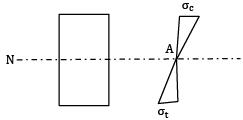Bending equation,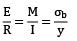σb ∝ y

y → Linear distance from neutral axis. Thus, bending stress is zero at neutral axis and maximum at outermost fibres.

QUESTION: 2

### The intensity of bending stress in the cross section at any point distance y from the neutral axis is proportional to (l is moment of inertia)

Solution: Bending equation,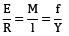f ∝ y

QUESTION: 3

### The bending stress calculated by using the formula M = fz is absolutely accurate when the bending moment1. Variation is linear2. Variation is parabolic (2nd degree)3. Is constant4. None of the above

Solution:
QUESTION: 4

A beam of uniform cross-section is subjected throughout its length to a uniform moment. Then the deflection shape of beam is

Solution:
QUESTION: 5

If E = elasticity modulus, I = moment of inertia about the neutral axis and M = bending moment in pure bending under the symmetric loading of a beam , the radius of curvature of the beam

1. increases with E

2. increases with M

3. Decreases with I

4. Decreases with M

Which of these are correct?

Solution: In pure bending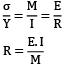R increases with E R decrease with M Statement 1 and 4 are correct.

QUESTION: 6

A mild steel beam is simply supported. It has a constant moment of inertia = 106 mm4. The entire length of the beam is subjected to a constant BM of 107 Nmm. E = 2 × 105 N/mm2. What is the radius curvature of the bent beam in meters?

Solution: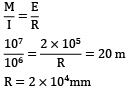QUESTION: 7

A rectangular bar of width b and depth ‘d’ is being used as a cantilever. The loading in a plane parallel to the side b. the section modulus is

Solution: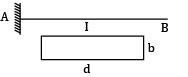Section modulus, Z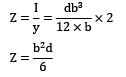QUESTION: 8

A beam is said to be uniform strength if

Solution:
QUESTION: 9

A rectangular section beam subjected to a bending moment M varying along its length is required to develop the same maximum bending stress at any cross-section. If the depth of the section is constant, then its width will vary as

Solution: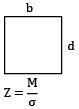Where, σ is the maximum bending stress at any cross-section throughout its length. If depth is also constant then b ∝ M

QUESTION: 10

Wire of diameter ‘d’ is wound round a cylinder. The diameter of the cylinder is ‘D’. The bending stress (maximum) induced in the wire is

Solution: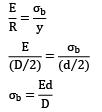QUESTION: 11

For a rectangular section beam, if the beam depth is doubled, keeping the width, length and loading same, the bending stress is decreased by a factor

Solution: For rectangular section,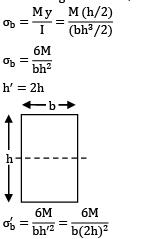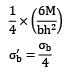QUESTION: 12

The ratio of width to depth of a strongest beam that can be cut out of a cylindrical log of wood is

Solution: Let D be dia of cylinder, b & t be width and depth of the beam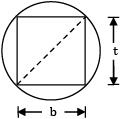D2 = b2 + t2 t2

= (D2 − b2) We have,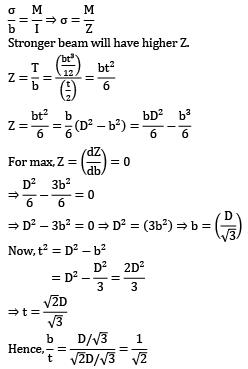Stronger beam will have higher Z.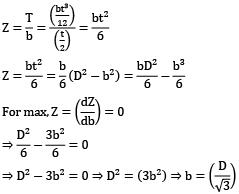ow, t2 = D2 − b2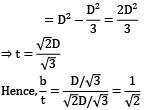QUESTION: 13

In a beam of circular cross-section, the shear stress variation due to shear force across a cross-section is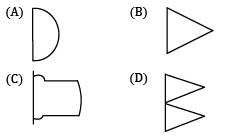Solution: Shear stress distribution in a circular section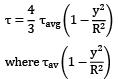Where V is shear force at section A is area of cross section.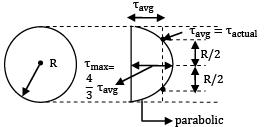QUESTION: 14

In a beam of solid circular cross-section, what is the ratio of maximum shear stress to the average shear stress?

Solution: Beam of circular section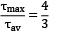QUESTION: 15

What is the nature of distribution of shear stress in a rectangular beam?

Solution: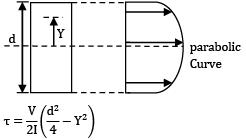QUESTION: 16

A simply supported beam of rectangular cross-section is under transverse loading. Regarding the shear stress distribution across any section, the ratio of maximum shear stress to mean shear stress is

Solution: For rectangular cross-section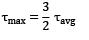QUESTION: 17

In case of a beam of I-section subjected to transverse shear force ‘F’, the maximum shear stress occurs the

Solution: I-section beam τmax occurs at the centre of the web.

QUESTION: 18

The distribution of shear stress of a beam is shown in the given figure.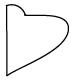The cross-section of the beam is

Solution: For T-section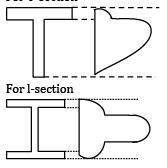QUESTION: 19

The shear stress distribution over a beam cross-section is shown in the figure below. The beam is of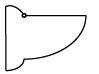Solution: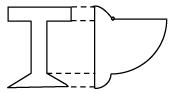QUESTION: 20

A cantilever is loaded by a concentrated load P at the free end as shown. The shear stress in the element LMNOPQRS is under consideration. This of the following figure represents the shear stress directions cantilever?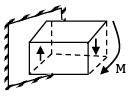Solution: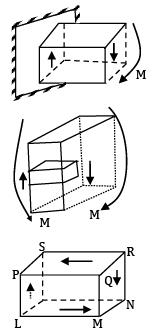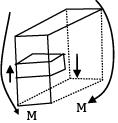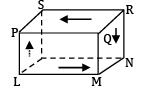The direction of shear stress will be as shown in option (A).